# 978-0134292380 Chapter 5 Part 2

Document Type
Test Prep
Book Title
Fundamentals of Hydraulic Engineering Systems 5th Edition
Authors
A. Osman H. Akan, Ned H. C. Hwang, Robert J. Houghtalen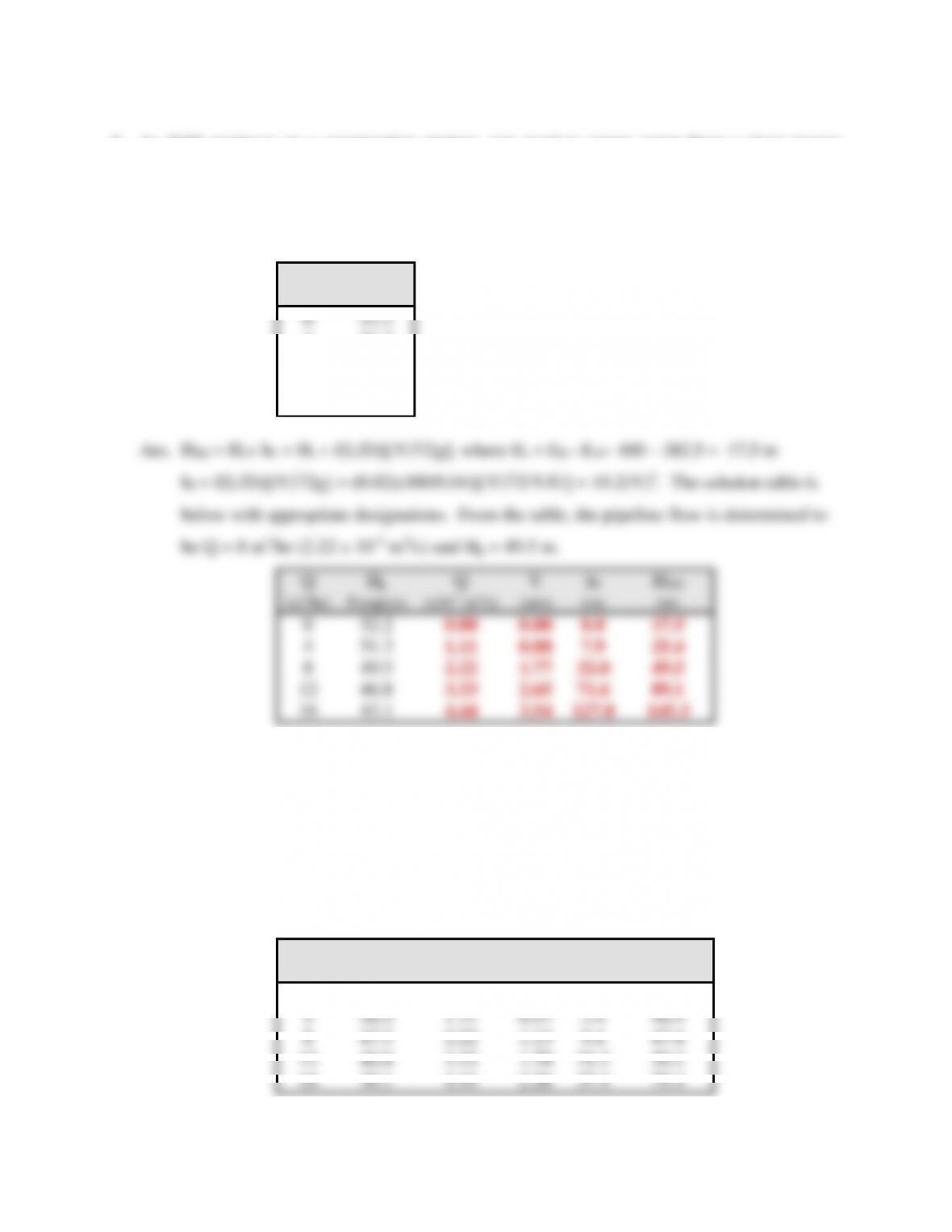49
6. As field engineer on a construction project, you need to pump water from a river (water
surface elevation of 382.5 meters) to a reservoir (water surface elevation 400 meters). The
pump available to you has the pump characteristics tabulated below. The pipeline will be 400
meters long, and you would like to use a 4-cm-diameter ductile iron pipe (f = 0.020). What
flow rate can be expected if minor losses are ignored.
Q
Hp
(m3/hr)
Pump(m)
52.2
4
51.3
8
49.5
12
46.8
16
43.1
25.4
49.5
49.5
12
46.8
3.33
89.1
16
43.1
4.44
7. As field engineer on a construction project, you need to pump water from a river (water
surface elevation of 362 meters) to a supply reservoir (water surface elevation 400 meters).
The available pump has the performance characteristics tabulated below. The discharge
pipeline is a 5-cm-diameter ductile iron pipe (f = 0.018) and is 400 meters long. You have
performed the calculations in the table below and you note that the likely flow rate is 8 m3/hr,
which is too low. To increase the flow rate, you may have access to an identical pump and
can hook it up in parallel to the existing pump, sending the increased flow through the same
pipeline. What is the flow rate that can be expected if minor losses are ignored?
Q
Hp
Q
V
hf
HSH
(m3/hr)
Pump(m)
(x10-3 m3/s)
(m/s)
(m)
(m)
0
51.6
0.00
0.00
0.0
38.0
4
1.11
40.4
47.5
2.22
1.13
9.4
47.4
12
40.8
3.33
1.70
59.2
16
30.1
4.44
2.26
75.4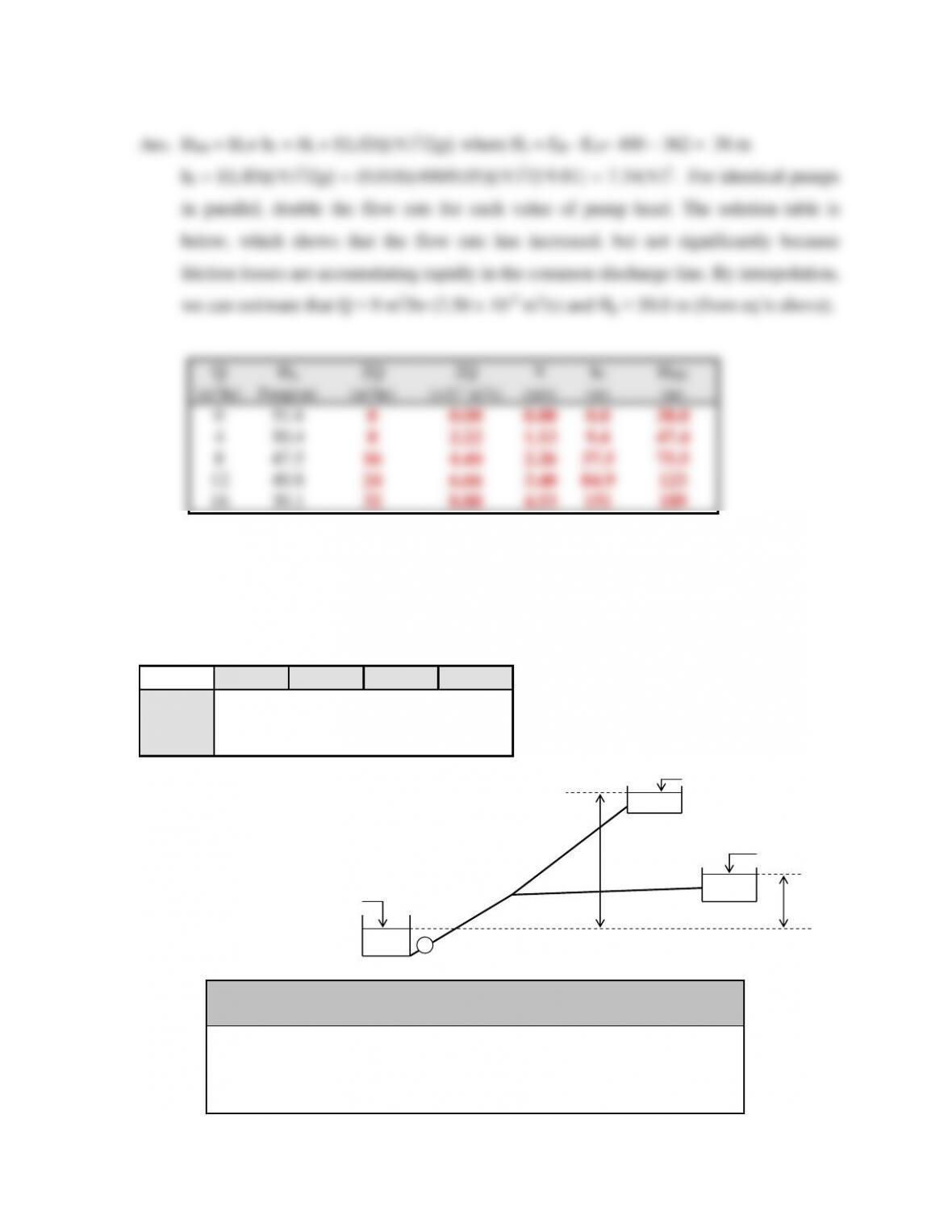50
84.9
123
16
30.1
32
8.88
4.53
151
8. Each of the three pipe flows is required in the branching pipe system depicted below. Fill in
the blanks of the solution table provided which eventually leads to the graphical solution.
The reservoir water elevations are: EA = 110 ft, EB = 120 ft, and EC = 140 ft.
Pipe Characteristics
Pipe
L (ft)
D (ft)
K
m
1
10000
2
0.0512
2
2
15000
2
0.0768
2
3
5000
2.5
0.0103
2
Q
Hp, ft
hf3
Net Hp*
HSH1
HSH2
(cfs)
(pump)
(ft)
(ft)
(ft)
(ft)
0.0
80.0
10.0
78.5
20.0
74.0
30.0
66.5
P
EA
EB
Hs1
EC
Hs2
Pipe 1
Pipe 2
Pipe 3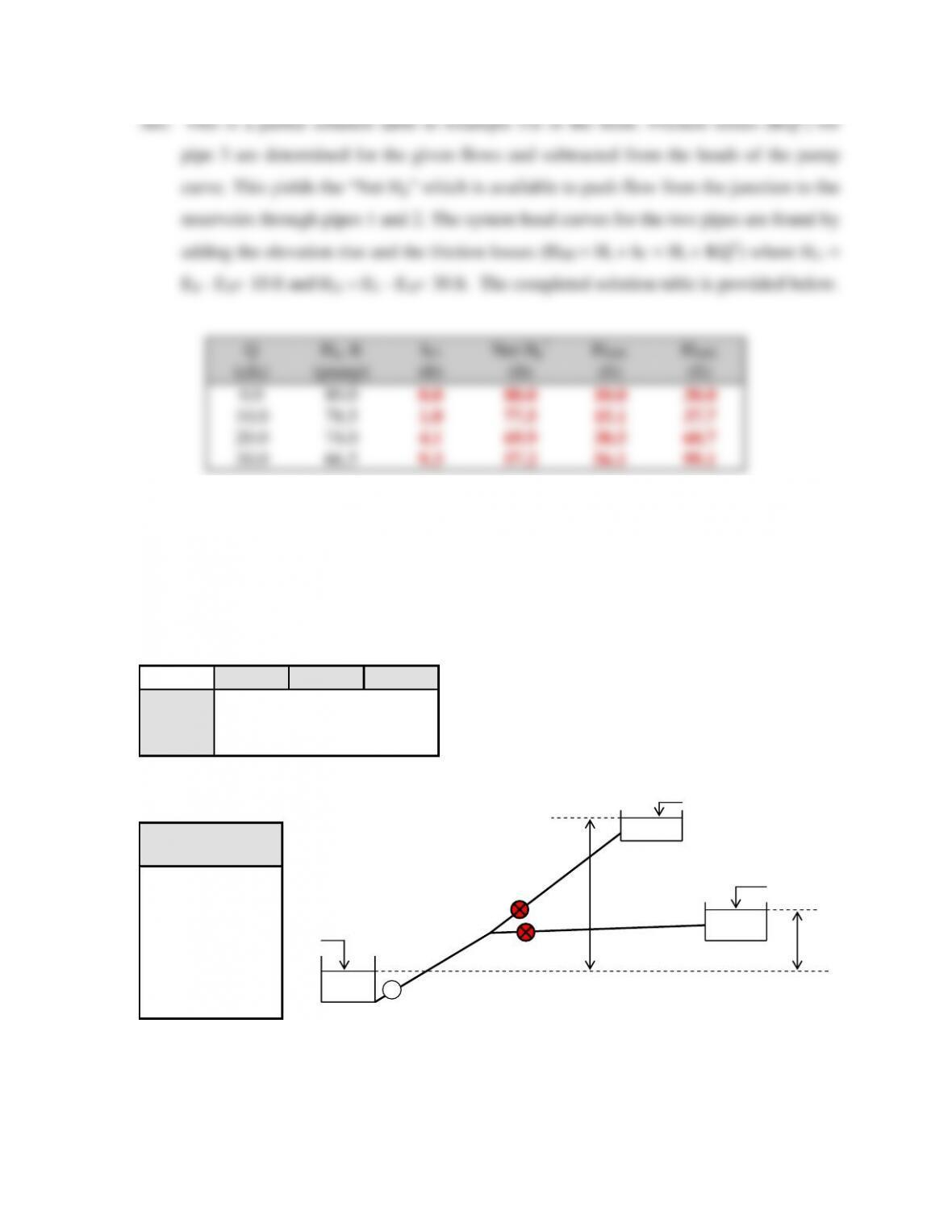51
9. The branching pipeline shown below contains a pump and three pipes. The characteristics of
the pipes and pump are tabulated below. Pipes 1 and 2 contain partially closed valves. If a
flow of 10 cfs is required to flow into Reservoir B and 10 cfs into Reservoir C, determine the
head loss in each valve. The reservoir water elevations are: EA = 100 ft, EB = 128 ft, and EC =
131 ft.
Pipe Characteristics
Pipe
L (ft)
D (ft)
f
1
1000
1.5
0.02
2
1000
1.5
0.02
3
1000
1.5
0.02
Q
Hp
(cfs)
Pump (ft)
0
100
5
96
10
90
15
80
20
66
25
48
30
24
P
EA
EB
Hs1
EC
Hs2
Pipe 1
Pipe 2
Pipe 3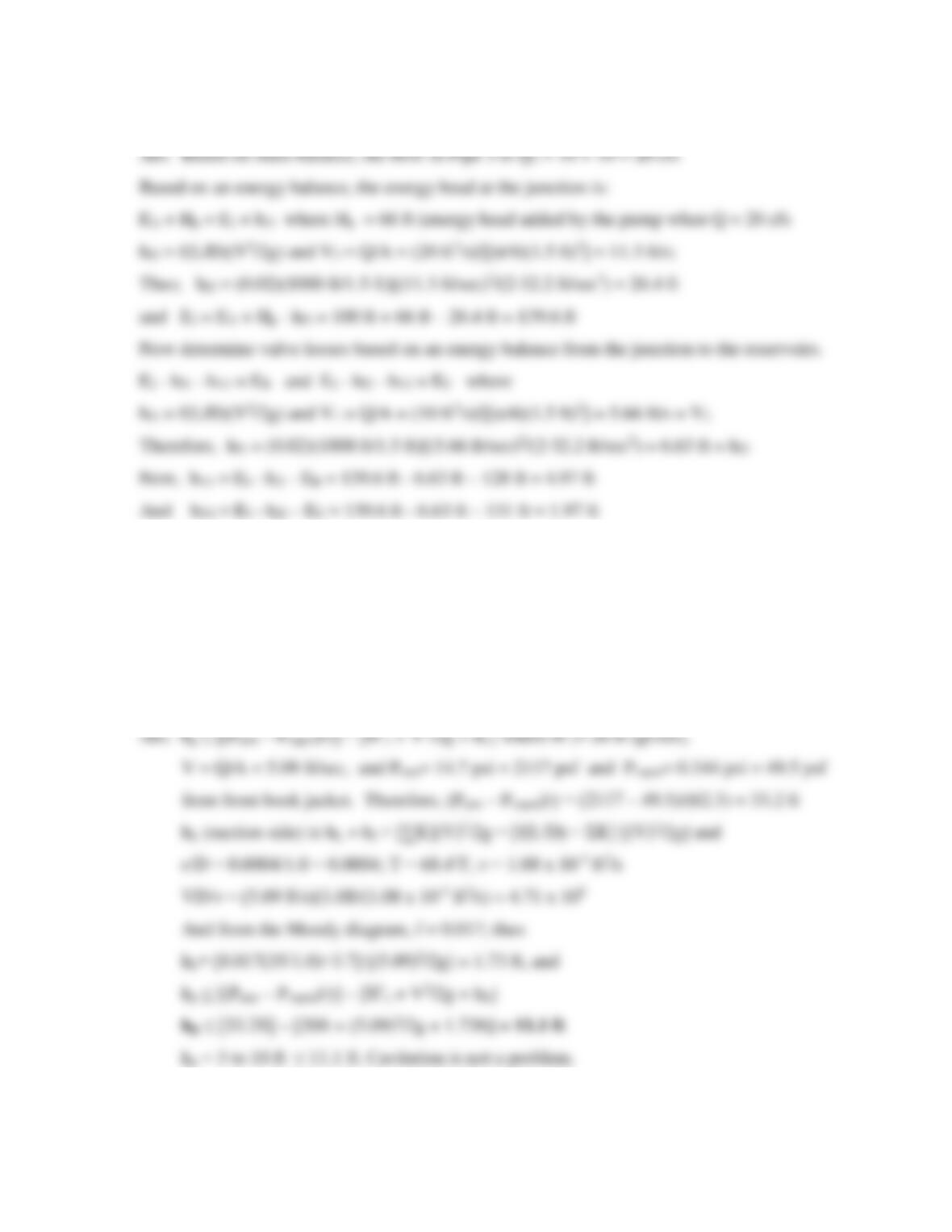52
10. A pump is installed in a 400-ft-long, 1.0-ft-diameter (ductile-iron) pipeline to deliver water
(68°F) from a supply reservoir to an elevated tank. The design discharge is 4.0 ft3/sec. The
pump overcomes an elevation difference between the reservoir and the tank of 150 ft. The
suction line is 35 ft of the 400-ft length. Minor loss coefficients in the suction line add up to
3.7. If the pump is installed 3 to 10 ft above the supply reservoir (depending on fluctuations
in the water level), will the installation be susceptible to cavitation? The net positive suction
head of the pump is rated at 20 ft.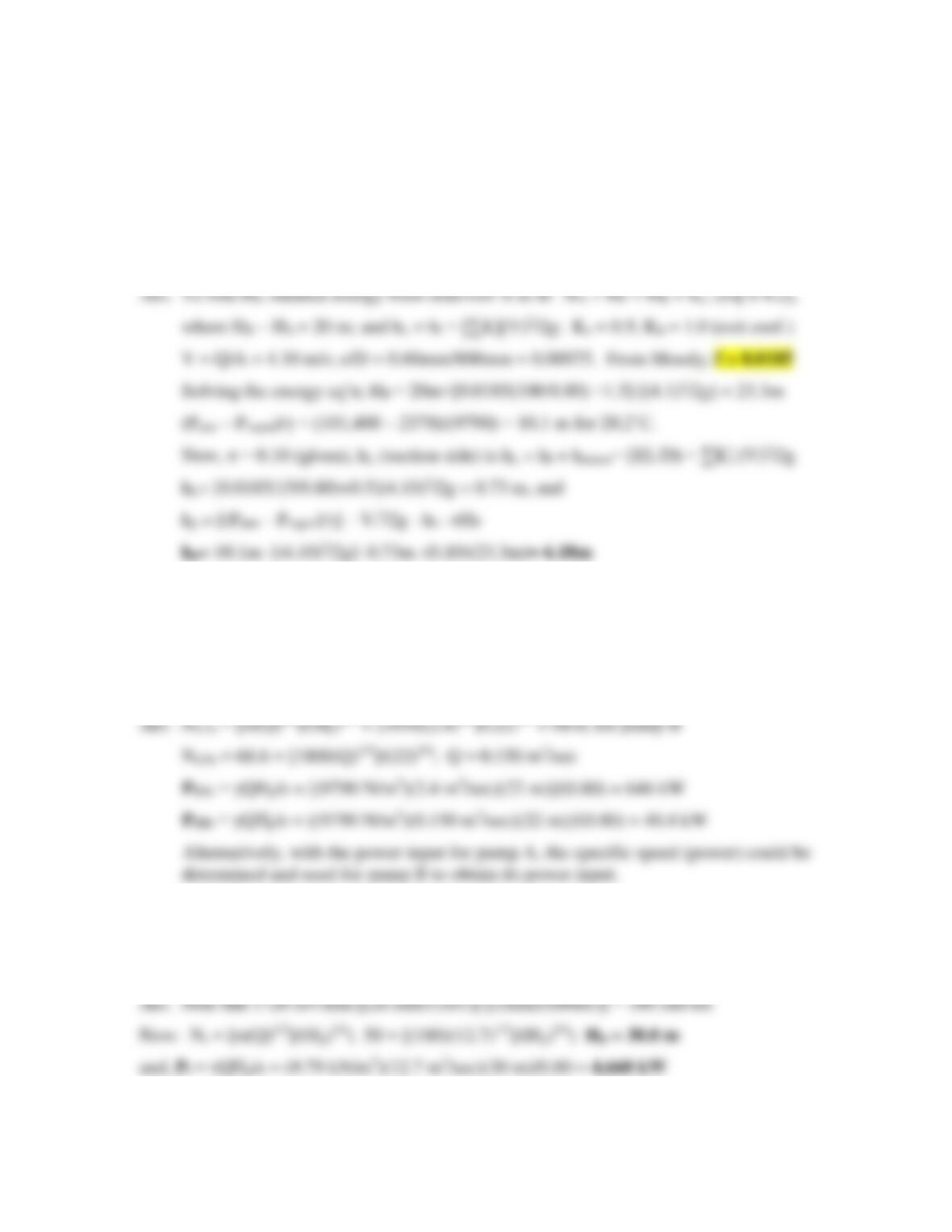53
11. A pump is installed in a 100-m pipeline to raise water (20° C) from reservoir A with a water
surface elevation of 145m to reservoir B with a water surface elevation of 165m. The 80-cm-
diameter, concrete (rough) pipe has a design discharge of 2.06 m3/sec. The suction line is 15
m of the 100-m length with a square-edged entrance condition. If the pump has a cavitation
parameter of 0.10, determine the allowable height the pump can be placed above the supply
reservoir to avoid cavitation. Assume completely turbulent flow in the pipeline.
12. Two geometrically-similar, centrifugal pumps are operated at the same efficiency. Pump A
operates at 450 rpm, and delivers 2.4 m3/sec against a 22-m head. The scale model (pump B)
is one fourth the size with corresponding dimensions and operates at four times the speed.
What is the discharge of pump B when it is delivering water against the same head? If the
efficiency of pump A is 0.80, determine the power input required of pump B.
13. A pump with a specific speed based on unit discharge of 50 has an impeller diameter of 72
cm, an operational speed of 1720 rpm, and an efficiency of 80%. Determine the pump head
and shaft power required to operate the pump if the discharge is 12.7 m3/sec.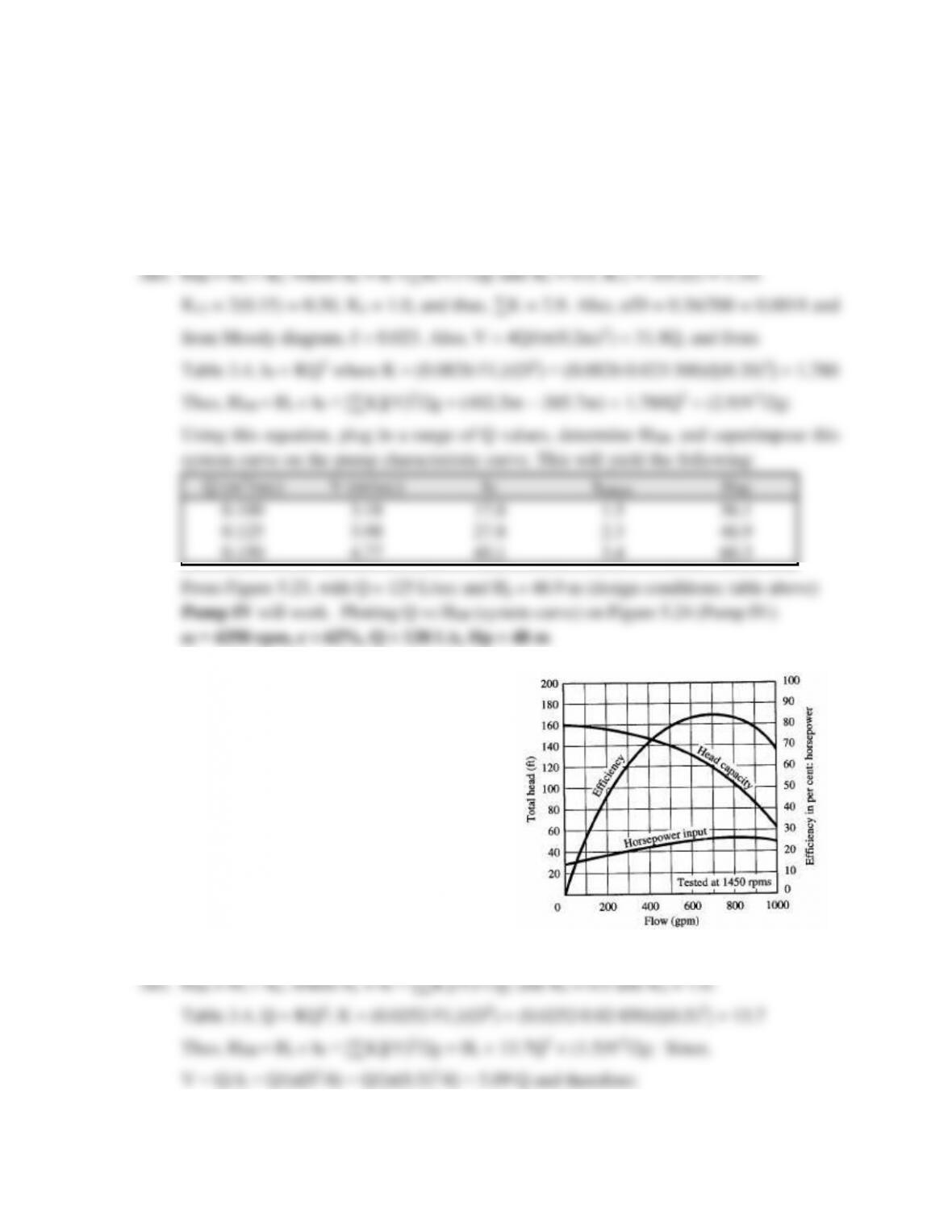54
14. A pump is required to deliver 0.125 m3/sec of water (β0˚C) from reservoir A to reservoir B
(water surface elevations of 385.7m and 402.5m, respectively). The pipeline (avg. concrete)
is 300 m long with a diameter of 0.20 m and contains five bends (R/D = 6) and two gate
valves. Determine the appropriate pump from Figure 5.23 and 5.24 and the pump’s working
conditions. Assume complete turbulence.
15. A pump, whose characteristic curve
is depicted is placed in a 6-in. dia.
pipeline, 850 ft long (f = 0.020) to
raise water from one reservoir to
another. The water surface elevation
difference between the reservoirs
fluctuates from 136 ft to 58 ft based
on water demands. Determine the
water surface elevation difference
between the supply and receiving
reservoirs when the pump is
delivering flow rates of 400 gpm,
600 gpm, and 800 gpm.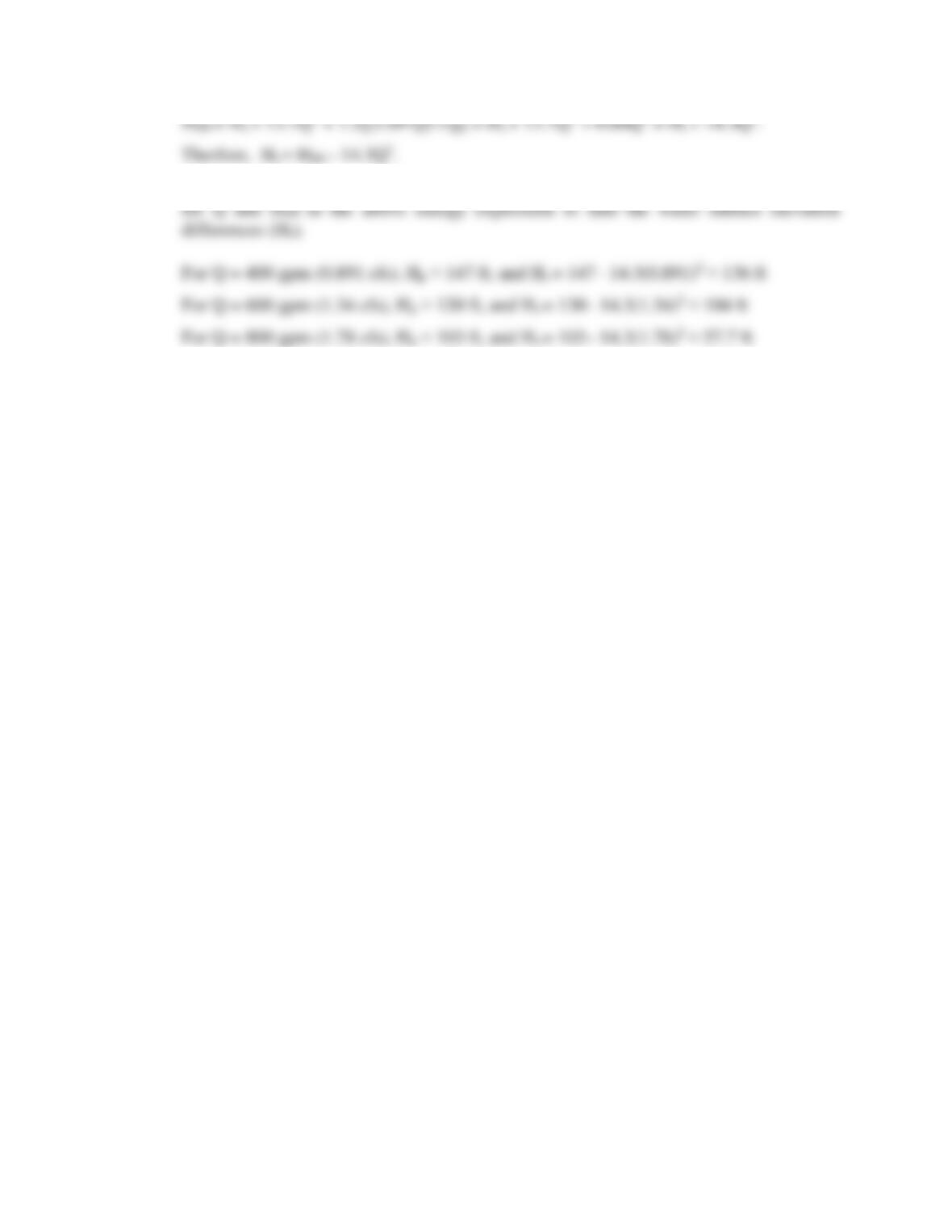43
For each Q, find Hp from the pump characteristic curve. Then substitute the Q and Hp

## Trusted by Thousands ofStudents

Here are what students say about us.